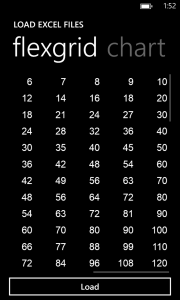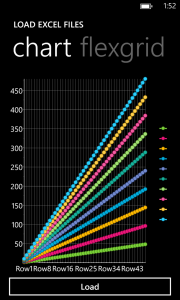Control libraries are extremely useful when you need to work with special file formats, like PDF and Excel, because learning how to read and write to these raw formats is very time consuming. ComponentOne has plenty of libraries and tools for these popular file formats including our Excel library for the Windows Phone. In this blog post I show how you can load Excel (XLSX) and CSV files using the C1Excel library, and then I show how you can display the contents of the file in a C1FlexGrid and C1Chart control.

## Reading a XLSX or CSV File

The ComponentOne Excel library for Windows Phone supports two file formats: XLSX (OpenXml) and CSV. It can also read OpenXML Template files. To read a file you simply call the Load method from the C1XLBook class. But first, let’s instantiate a new book and obtain a stream to some file.

``````
StreamResourceInfo sri = Application.GetResourceStream(new Uri("/<name of assembly>;component/Resources/Sample.xlsx", UriKind.Relative));
var book = new C1.Phone.Excel.C1XLBook();

``````

This code assumes that you have a file named Sample.xlsx (Build Action = Resource) in a Resources directory. If you wish to load a CSV file instead, simply change the FileFormat parameter in the Load method. Now we have loaded an Excel file so what next? The ComponentOne Excel library (namely the C1XLBook class) makes it easy to read and write to the file using basic C# or VB code. For instance, the following line of code sets a cell in sheet #0, row #1 and column #2 to the value of “3.”

``````
book.Sheets[1, 2].Value = "3";

``````

Next, I’ll use this basic principle to retrieve all the values from the file and load them into a C1FlexGrid control on my page.

## Displaying Excel Contents in Your App

Display using C1FlexGrid The block of code below will load a given sheet into a C1FlexGrid control. So if your workbook has multiple sheets then you’ll need to configure your UI to handle this (ie, multiple pivot items). The basic concept is to loop through the columns and rows copying each cell’s value into the FlexGrid control. I’ve left several lines of code uncommented out. These show additional options you can set based on your file.

``````
/// <summary>
/// Loads the content of an XLSheet into a C1FlexGrid.
/// </summary>
public static void LoadFlexGrid(XLSheet sheet, C1FlexGrid flex)
{
// set default parameters
flex.FontFamily = new FontFamily(sheet.Book.DefaultFont.FontName);
flex.GridLinesVisibility = sheet.ShowGridLines
? GridLinesVisibility.All
: GridLinesVisibility.None;
flex.GridLinesBrush = new SolidColorBrush(sheet.GridColor);

flex.Columns.Clear();
foreach (XLColumn c in sheet.Columns)
{
// create column
var col = new Column();
//col.ColumnName = col.GetHashCode().ToString("x0");
//col.Header = "ds " + (flex.Columns.Count + 1).ToString();
col.Visible = c.Visible;

// and add to the grid
}

flex.Rows.Clear();
foreach (XLRow r in sheet.Rows)
{
var row = new Row();
row.Visible = r.Visible;
}

for (int r = 0; r < flex.Rows.Count; r++)
{
for (int c = 0; c < flex.Columns.Count; c++)
{
var cell = sheet[r, c];
if (cell != null)
{
if (cell.Value != null)
{
flex[r, c] = cell.Value;
}
}
}
}

// freeze rows/columns
flex.Rows.Frozen = sheet.Rows.Frozen;
flex.Columns.Frozen = sheet.Columns.Frozen;
}

``````Display using C1Chart The following block of code will load the contents of the workbook into a C1Chart control. When using a chart the requirements are a bit more strict because you need rows and columns of numbers specifically. This code will create a new data series for each column of the excel file. Then it loops through each row adding the values to each series. Finally, it will create a list of string (item names) to display along the x-axis. The original Excel file does not contain a label for each row, however this code could be extended to retrieve the first column of labels to display along the x-axis.

``````
/// <summary>
/// Loads the content of an XLSheet into a C1FlexGrid.
/// </summary>
public static void LoadChart(XLSheet sheet, C1Chart chart)
{
chart.Reset(true);
int r = 0;
for (int c = 0; c < sheet.Columns.Count; c++)
{
// add a data series for each column
DataSeries ds = new DataSeries();
//ds.Label = "Col" + (c + 1).ToString();
ds.Values = new DoubleCollection();
for (r = 0; r < sheet.Rows.Count; r++)
{
// add value for each row
var cell = sheet[r, c];
double value;
if (cell.Value != null)
{
if (double.TryParse(cell.Value.ToString(), out value))
{
}
}
}

}

// creat list of item names along x axis. in this case these are row numbers.
List<string> itemNames = new List<string>();
for (int i = 1; i < r; i++)
{
}
chart.Data.ItemNames = itemNames;
}

``````To load the first sheet from you workbook into an existing C1Flexgrid and C1Chart on your page, the code would look like this:

``````
if (book.Sheets.Count > 0)
{